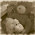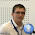### Compare two person name (Are the names similar?)

Working on a personal project I need a mechanism to compare two names and find out if they are similar. The first think that I done was to try to define a regular expression that could help me.
I end up studying different algorithms that can measure the distance between two sequences.  In this post I will talk about what algorithms I found and what I finally used.
First algorithm that I found was the based on Hammed distance. This algorithm measure the minimum number of substitutions that need to be done to change a string to another string. This is a great algorithm that works very well when we have string with the same length.
Another interesting algorithm was based on Euclidean distance and is used to measure the distance between two points. It is based on Pythagorean formula. It is a pretty complicated algorithm and in my case was too complicated. I need a simple solution, that doesn't consume a lot of resources. I don’t need exact match each time.
The 3th algorithm that I discover was Jaccard similarity coefficient. Using this solution you can obtain the similarity and diversity value of two sets – in our case two strings.
In the end I found what I need. The Levenshtein distance compare two sequences and measure the difference between them. It will give us how many changes we need to make to the first sequence to obtain the second one.
It is pretty similar to Hammed algorithm, but in my case this solution is good and resolves my problem. The algorithm returns a positive number. In my case when the returned value is 2 or lower I consider that the two names are similar.
Of course there are also a lot of solutions, different components that resolve this problem. In my case this was the perfect solution. The implementation in C# can be found at the end of the post.
You will observe that the algorithm is recursive. We can rewrite it in different ways of course.
Recursive version:
``````int LevenshteinDistance(string s, string t)
{
int n = s.Length;
int m = t.Length;

if (n == 0)
{
return m;
}
if (m == 0)
{
return n;
}

int cost = s[n - 1] == t[m - 1]
? 0
: 1;

return
Math.Min(
Math.Min(LevenshteinDistance(s.Substring(0, n - 1), t) + 1,
LevenshteinDistance(s, t.Substring(0, m - 1)) + 1),
LevenshteinDistance(s.Substring(0, n - 1), t.Substring(0, m - 1)) + cost);
}
``````
Non recursive version:
``````int LevenshteinDistance(string s, string t)
{
int n = s.Length;
int m = t.Length;
int[,] d = new int[n + 1, m + 1];

if (n == 0)
{
return m;
}

if (m == 0)
{
return n;
}

for (int i = 0; i <= n; d[i, 0] = i++){ }
for (int j = 0; j <= m; d[0, j] = j++){ }

for (int i = 1; i <= n; i++)
{
for (int j = 1; j <= m; j++)
{
int cost = (t[j - 1] == s[i - 1])
? 0
: 1;
d[i, j] = Math.Min(
Math.Min(
d[i - 1, j] + 1,
d[i, j - 1] + 1),
d[i - 1, j - 1] + cost);
}
}
return d[n, m];
}
``````

1.FYI: Levenshtein distance can be implemented with 2*min(n,m) storage space rather than n*m (that is, you just need the previous and current row of the matrix, and LD(a,b) == LD(b,a) so you can choose the shorter word as the row length).

Also, for English-only names / words you can use SoundEx: http://en.wikipedia.org/wiki/Soundex

1.There is also the double-megaphone search algorithm.
The code is not optimized, is only a sample code.

### ADO.NET provider with invariant name 'System.Data.SqlClient' could not be loaded

Today blog post will be started with the following error when running DB tests on the CI machine:
threw exception: System.InvalidOperationException: The Entity Framework provider type 'System.Data.Entity.SqlServer.SqlProviderServices, EntityFramework.SqlServer' registered in the application config file for the ADO.NET provider with invariant name 'System.Data.SqlClient' could not be loaded. Make sure that the assembly-qualified name is used and that the assembly is available to the running application. See http://go.microsoft.com/fwlink/?LinkId=260882 for more information. at System.Data.Entity.Infrastructure.DependencyResolution.ProviderServicesFactory.GetInstance(String providerTypeName, String providerInvariantName) This error happened only on the Continuous Integration machine. On the devs machines, everything has fine. The classic problem – on my machine it’s working. The CI has the following configuration:

TeamCity.NET 4.51EF 6.0.2VS2013
It seems that there …

### Entity Framework (EF) TransactionScope vs Database.BeginTransaction

In today blog post we will talk a little about a new feature that is available on EF6+ related to Transactions.
Until now, when we had to use transaction we used ‘TransactionScope’. It works great and I would say that is something that is now in our blood.
using (var scope = new TransactionScope(TransactionScopeOption.Required)) { using (SqlConnection conn = new SqlConnection("...")) { conn.Open(); SqlCommand sqlCommand = new SqlCommand(); sqlCommand.Connection = conn; sqlCommand.CommandText = ... sqlCommand.ExecuteNonQuery(); ... } scope.Complete(); } Starting with EF6.0 we have a new way to work with transactions. The new approach is based on Database.BeginTransaction(), Database.Rollback(), Database.Commit(). Yes, no more TransactionScope.
In the followi…

### GET call of REST API that contains '/'-slash character in the value of a parameter

Let’s assume that we have the following scenario: I have a public HTTP endpoint and I need to post some content using GET command. One of the parameters contains special characters like “\” and “/”. If the endpoint is an ApiController than you may have problems if you encode the parameter using the http encoder.
using (var httpClient = new HttpClient()) { httpClient.BaseAddress = baseUrl; Task<HttpResponseMessage> response = httpClient.GetAsync(string.Format("api/foo/{0}", "qwert/qwerqwer"))); response.Wait(); response.Result.EnsureSuccessStatusCode(); } One possible solution would be to encode the query parameter using UrlTokenEncode method of HttpServerUtility class and GetBytes method ofUTF8. In this way you would get the array of bytes of the parameter and encode them as a url token.
The following code show to you how you could write the encode and decode methods.
publ…